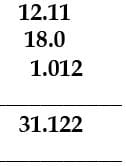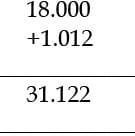# SIGNIFICANT FIGURES Notes - Class 11

## Class 11: SIGNIFICANT FIGURES Notes - Class 11

The document SIGNIFICANT FIGURES Notes - Class 11 is a part of Class 11 category.
All you need of Class 11 at this link: Class 11

SIGNIFICANT FIGURES
The reliability of a measurement is indicated by the number of digits used to represent it. To express the measurement more accurately we express it with digits that are known with certainty. These are called as Significant figures. They contain all the certain digits plus one doubtful digit in a number.

RULES FOR DETERMINING THE NUMBER OF SIGNIFICANT FIGURES

• All non-zero digits are significant. For example, 6.9 has two significant figures, while 2.16 has three significant figures.
• The decimal place does not determine the number of significant figures.
• A zero becomes significant in case it comes in between non zero numbers. For example, 2.003 has four significant figures, 4.02 has three significant figures.
• Zeros at the beginning of a number are not significant. For example, 0.002 has one significant figure while 0.0045 has two significant figures.
• All zeros placed to the right of a number are significant. For example, 16.0 has three significant figures, while 16.00 has four significant figures.
• Zeros at the end of a number without decimal point are ambiguous.
• In exponential notations, the numerical portion represents the number of significant figures. For example, 0.00045 is expressed as 4.5 x 10-4 in terms of scientific notations. The number of significant figures in this number is 2, while in Avogadro's number (6.023 x 1023) it is four.
• The decimal point does not count towards the number of significant figures. For example, the number 345601 has six significant figures but can be written in different ways, as 345.601 or 0.345601 or 3.45601 all having same number of significant figures.

MATH WITH SIGNIFICANT FIGURES

(i) ADDITION AND SUBTRACTION OF SIGNIFICANT FIGURES
The result cannot have more digits to the right of the decimal point than either of the original numbers. 12.11
18.0
1.012
31.122
Here, 18.0 has only one digit after the decimal point and the result should be reported only up to one digit after the decimal point, which is 31.1.

(ii) MULTIPLICATION AND DIVISION OF SIGNIFICANT FIGURES
In these operations, the result must be reported with no more significant figures as in the measurement with the few significant figures.
2.5 × 1.25 = 3.125
Since 2.5 has two significant figures, the result should not have more than two significant figures, thus, it is 3.1.

PRACTICE QUESTIONS
Q.1. How many Significant figures in each term?
(a) 34.6209
(b) 0.003048
(c) 5010.0
(d) 4032.090
Ans.
(a) 6
(b) 4
(c) 5
(d) 7

Q.2. Solve the following equations using the correct number of significant figures.
(a) 34.683 + 58.930 + 68.35112
(b) 45001 - 56.355 - 78.44
(c) 0.003 + 3.5198 + 0.0118
(d) 36.01 - 0.4 - 15

Ans.
(a) 161.964
(b) 44866
(c) 3.535
(d) 21

Q.3. Solve the following equations using the correct number of significant figures.
(a) 98.1 x 0.03
(b) 57 x 7.368
(c) 8.578 / 4.33821
(d) 6.90 / 2.8952

Ans.
(a) 3
(b) 4.2 x 102
(c) 1.977
(d) 2.38

Q.4. How many significant figures in each term?
(a) 1.40 x 103
(b) 6.01
(c) 02947.1
(d) 583.02

Ans.
(a) 3
(b) 3
(c) 5
(d) 5

Try yourself!
Q.1. Look at the addition of significant figures given belowThe result reported in this addition should be
(a) 31.12
(b) 31.1
(c) 31.122
(d) 31.10
Ans. (b)
Solution. The result cannot have more digits to the right of the decimal point than either of the original numbers 12.110Here, 18.0 has only one digit after the decimal point and the result should be reported only upto one digit after the decimal point which is 31.1 .

Q.2. The result reported in the following multiplication of significant figures, 2.5 ×1.25 = 3.125 should be
(a) 3.125
(b) 3.1
(c) 3.12
(d) 3.10
Ans. (b)
Solution. In these operations, the result must reported with no more significant figures as are there in the measurement with the few significant figures.
2.5 × 1.25 = 3.125
Since, 2.5 has two significant figures, the result should not have more than two significant figures thus. It is 3.1.

Q.3. If 6.25 is to be rounded off it is A and if 6.35 is to be rounded off it is rounded off to B. Here , A and B refer to
(a) A → 6.2, B → 6.4
(b) A → 6.1, B → 6.3
(c) A → 6.3, B → 6.4
(d) A = 6.1, B = 6.2
Ans. (a)
Solution. If the rightmost digit to be removed is 5, then the preceding number is not changed if it is an even number but it is increased by one if it is an odd number, e.g. if 6.35 is to be rounded by removing 5, We have to increase 3 to 4 giving 6.4 as the result. However, if 6.25 is to be rounted off it is rounded off to 6.2.

The document SIGNIFICANT FIGURES Notes - Class 11 is a part of Class 11 category.
All you need of Class 11 at this link: Class 11Use Code STAYHOME200 and get INR 200 additional OFF

Track your progress, build streaks, highlight & save important lessons and more!

,

,

,

,

,

,

,

,

,

,

,

,

,

,

,

,

,

,

,

,

,

;